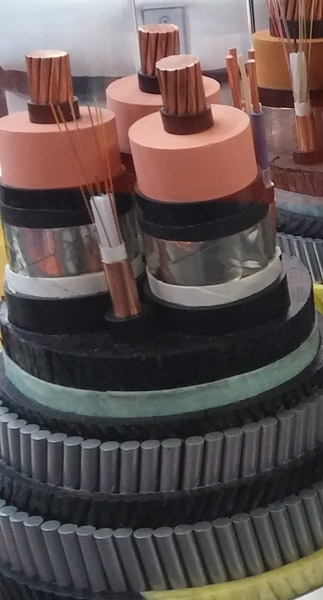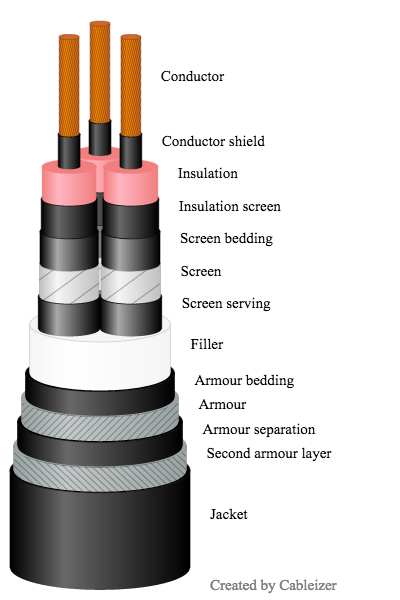# Two armour layers with separation

Calculating the ampacity of a cable system correctly starts with the precise modeling of the cable. The user shall be able to model a cable as close to reality as possible, visually, electrically and thermally. As a result from our participation at the Cigré 2016 in Paris, we have now introduced a separation layer between the two metallic armour layers.

Posted 2016-09-02
Categories: New feature, Theory

### New feature

We already introduced double armoured cables and wrote about it in a previous blog post. Now we extended this by giving the user the possibility to add a separation layer between the two metallic armour layers. This separation acts as a thermal resistance resulting in a temperature difference between the two metallic armour layers.

Cableizer participated at the Cigré 2016 in Paris where we were able to admire many beautiful cable models. A few models for submarine cables used a double armour and some had a separation layer with significant thickness in between. One of our goals is to give the user the possibility to model all types of cables the way they are actually built and to calculate them correctly. Therefore, we decided to add this separation layer to our modeling capability. This Thursday, less than one week later, this new feature is available for all users. At the same time, we also added flat copper wires for the armour because this was used in submarine cables from a Korean and a Japanese manufacturer.

The reason for this separation layer is due to manufacturing processes and not to separate the armour layers electrically. But since it creates a thermal barrier between the two layers, they will reach different temperatures and therefore have a different electrical resistance because it is temperature dependent. The difference is of course small, especially compared to other effects.

### Software model vs. reality

Cable sample from manufacturer 3D software model of the cable 2D software model of the cable (new)Please note that all elements in the 3D model are proportional. And since a few weeks, we also show a 2D model of the cables because many people were more used to this. Of course, all elements are also proportional in the 2D model.

### Calculation

The following formulas show how this double armour layer with separation is calculated.

The resistance of each layer is calculated separately using the corresponding dimensions and temperatures.

 $R_{Ao} \left(\alpha_{ar} \left(\theta_{ar 1} - 20\right) + 1\right)$ $R_{Ao 2} \left(\alpha_{ar} \left(\theta_{ar 2} - 20\right) + 1\right)$

The temperatures of the two metallic armour layers are calculated as follows:

 $- T_{1} \left(I_{c}^{2} R + \frac{W_{d}}{2}\right) - T_{2 1} n_{c} \left(I_{c}^{2} R \left(\lambda_{1} + 1\right) + W_{d}\right) + \theta_{c}$ $- T_{1} \left(I_{c}^{2} R + \frac{W_{d}}{2}\right) - T_{2} n_{c} \left(I_{c}^{2} R \left(\lambda_{1} + 1\right) + W_{d}\right) + \theta_{c}$

The $T_2$ in the second formula is the sum of the two thermal resistivities $T_{2_1}$ and $T_{2_2}$. The calculation of $θ_{ar_2}$ therefore neglects the heat losses generated in the first armour layer that lies below the separation layer. However, this leads to only a small deviation which is acceptable, especially considering the fact, that the armour losses calculated with the IEC method are usually too high, a fact which was also presented in several publications at Cigré.

The armour temperature is the mean value of the temperatures of the first and second armour layer. Because the resistances of the armour layers are calculated based on the temperatures of the corresponding layers, this temperature is not used but for information only.

 $\frac{\theta_{ar 1}}{2} + \frac{\theta_{ar 2}}{2}$

with the thermal resistance between sheath and first armour layer and between the first and second armour layers:

 $\frac{G_{2} \rho_{f}}{6 \pi} + \frac{\rho_{2}}{2 \pi} \log{\left (\frac{2 t_{ab}}{d_{A 1} - d_{f} - 2 t_{ab}} + 1 \right )}$ $\frac{\rho_{2 2}}{2 \pi} \log{\left (1 + \frac{2}{D_{a}} t_{ab 2} \right )}$

6
25
12
24
10
9
10
2
9
11
2